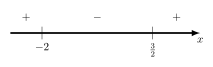# 1.2b. Solving a rational inequality algebraically

Example question: Solve algebraically.

As shown in the previous example , we solve an inequality by first making one side $0$ (which is already true for this example) and then trying to factorize everything. Unfortunately, $x^2+2x+3$ cannot be factorized for this question. So how do we proceed?

$\displaystyle \frac{(x+1)^2 + 2}{2x^2+x-6} < 0$
Since $(x+1)^2 \geq 0$ for all $x \in \mathbb{R}$, $(x+1)^2 + 2$ is always positive for all real $x$.
Hence $\displaystyle \frac{1}{2x^2+x-6} > 0$
$\displaystyle \frac{1}{(x+2)(2x-3)} > 0$Why can't we factorize a quadratic expression?

We will look at 3 expressions, $x^2-x-6$, $x^2 + x - 1$ and $x^2 + x + 1$.

For the first case $x^2-x-6$, we are able to factorize the expression into $(x+2)(x-3)$. This gives us the roots $-2$ and $3$ which can be used in the number line method. This technique is illustrated in the previous example .

For the second case $x^2+x-1$, we are unable to factorize using only integers because the roots, in exact form, contain surds. We can obtain the roots using the quadratic formula $\displaystyle \frac{-b \pm \sqrt{b^2-4ac}}{2a}$ nevertheless. This allows us to factor $x^2+x-1$ into $\left (x - \frac{-1 - \sqrt{5}}{2} \right ) \left (x - \frac{-1 + \sqrt{5}}{2} \right)$ which allows us to proceed just like in the previous example .

Finally, $x^2+x+1$ is not factorizable because it has no real roots and is always positive. The next two sections explain what to do for such a case.

Step 2b.1: If a quadratic has no real roots, complete the square to show that it is always positive/negative

We note that $x^2 + 2x + 3$ has no real roots and is always positive. To explain this clearly, we complete the square to obtain $(x+1)^2 + 2$. Perfect squares like $(x+1)^2$ cannot be negative so the addition of 2 makes the entire expression always positive regardless of what $x$ is. This explains the first two lines of the worked solution above.

We can use the same approach to handle expressions like $-x^2-2x-2 = -(x+1)^2-1$ which is always negative. In this case, however, remember to flip the sign of the inequality when carrying out the multiplication/division step.

Step 2b.2: Now that the expression is always positive/negative, multiply/divide by it

Recall the rule for multiplication/division involving inequalities: keep the sign if the term we multiply/divide by is positive, and flip the sign if we multiply/divide by a negative term. We have warned against cross multiplication because most algebraic terms are sometimes positive or negative depending on what $x$ is. For this example, however, $(x+1)^2 + 2$ is always positive so we can move it to the right side of our expression. $0$ divided by $(x+1)^2 + 2$ is still $0$, which explains how we arrive at $\displaystyle \frac{1}{(x+2)(2x-3)} > 0$.

Step 3: Obtain the answer with the number line method

We complete the question with the number line approach as explained in the previous example .